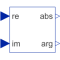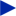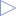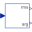# RectangularToPolar

Convert rectangular coordinates to polar coordinates# Information

This information is part of the Modelica Standard Library maintained by the Modelica Association.

The input values of this block are the rectangular components `u_re` and `u_im` of a phasor in two dimensions. This block calculates the length `y_abs` and the angle `y_arg` of the polar representation of this phasor.

```  y_abs = abs(u_re + j*u_im) = sqrt( u_re2 + u_im2 )
y_arg = arg(u_re + j*u_im) = atan2(u_im, u_re)
```

# Connectors (4)

u_re u_imType: RealInput Description: Real part of rectangular representationType: RealInput Description: Imaginary part of rectangular representationType: RealOutput Description: Length of polar representationType: RealOutput Description: Angle of polar representation

# Used in Components (1)Harmonic Modelica.Blocks.Math Calculate harmonic over period 1/f